Okay, we are plotting sine waves. What we do is we add a bunch of sinewaves together. The sinewaves are all clustered around a central frequency (1.0) in such a way as to fit a normal distribution curve (bell curve).

So here is the normal distrbution for one sine wave. You see there is only one frequency, and the resulting sinewave looks as you would expect.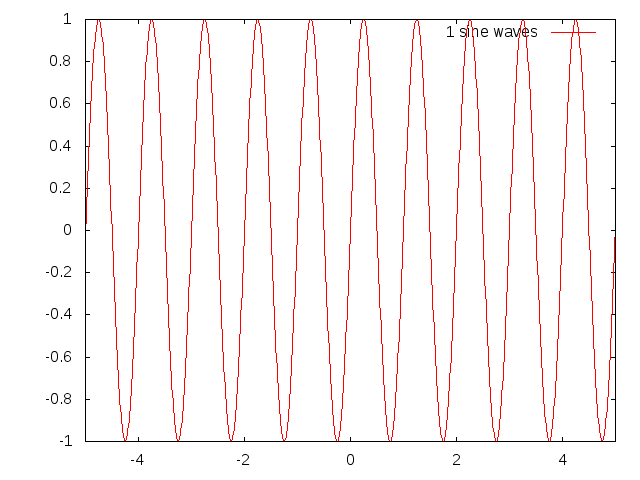So here is one with 3 sinewaves...one at the central frequency and one on either side. It demonstrates a beat note: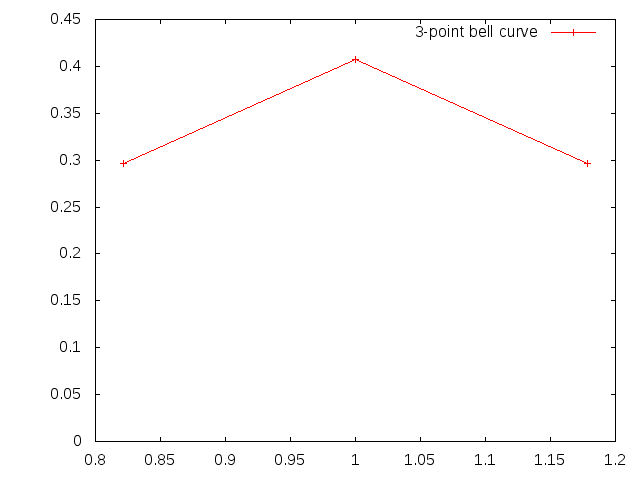Now here is 9 sinewaves, 4 on either side of the central frequency. Observe the X scale: the beats are further apart: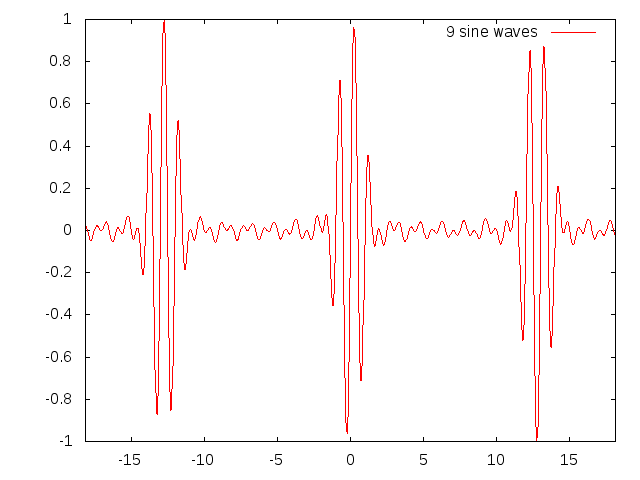19 sinewaves:39 sinewaves: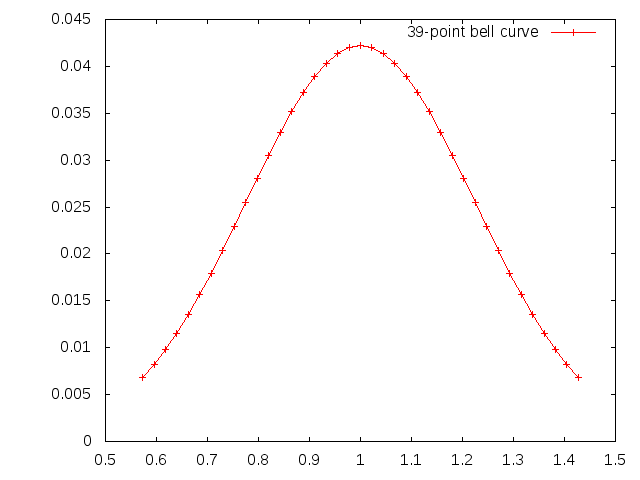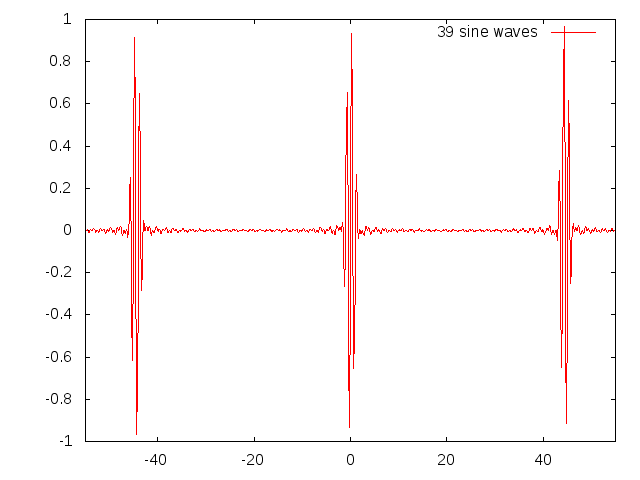199 sinewaves: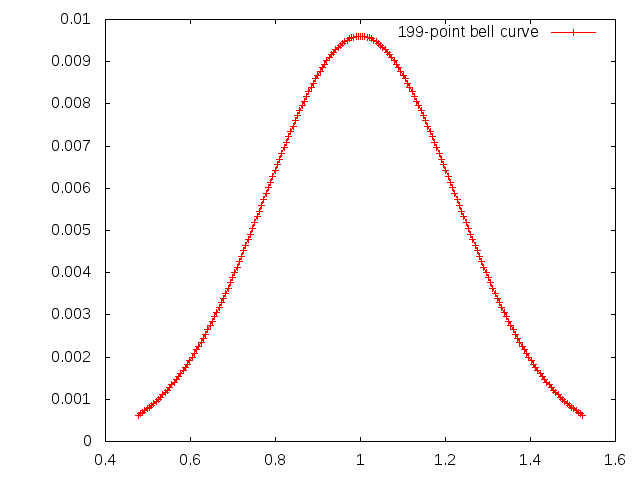1999 sinewaves: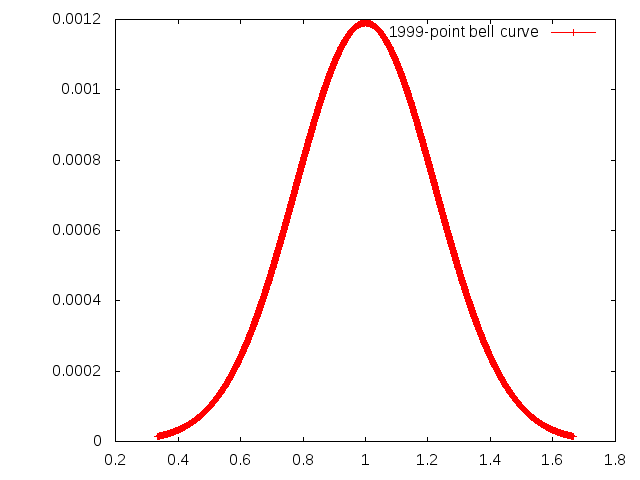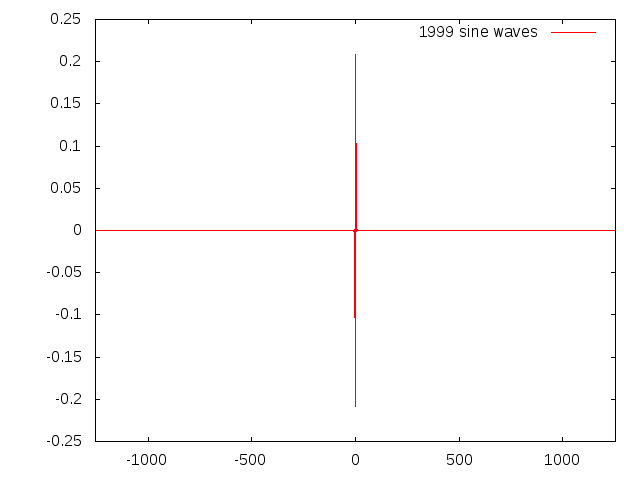If there are an infinite number of sinewaves clustered in a normal distribution around a single frequency, then the space between the beat notes is infinite: there is only a single pulse at zero.

The implication is that some fundamental thing that we think of as quantized (perhaps an electron) is actually the result of an interference pattern between a nearly infinite number of waves combining to make a beat that appears to be a single point.